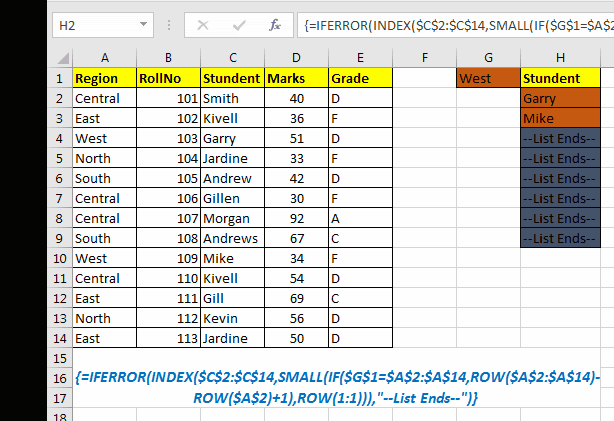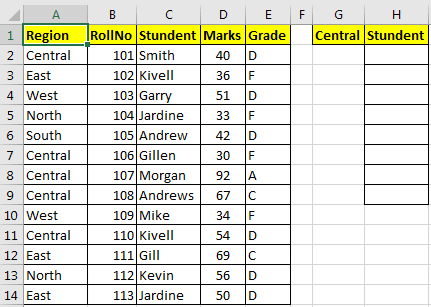# How to lookup multiple instances of a valueIn this article, we will learn How to look up multiple instances of a value in Excel.

Lookup values using the drop down option?

Here we understand how we can look up different results using the INDEX function array formula. Just select the value from the list and the corresponding result will be there.

Generic Formula

 {=INDEX(array,SMALL(IF(lookup_value=lookup_value_range,ROW(lookup_value_range)-ROW(first cell of lookup_value_range)+1),ROW(1:1)))}

Array: The range from where you want to fetch data.

lookup_value: Your lookup_value that you want to filter.

lookup_value_range: The range in which you want to filter lookup_value.

The first cell in lookup_value range: if your lookup_value range is \$A\$5:\$A\$100 then its \$A\$5.

Important: Everything should be absolute referenced. lookup_value can be relative according to requirement.

Enter it as an array formula. After writing the formula use CTRL+SHIFT+ENTER key to make it an array formula.

### Example of lookup for multiple results

I have this student data in range A2:E14. In cell G1, I have a drop-down of region values e.g. Central, East, North, South, and West. Now I want, whichever region I have in G1, a list of all students from that region should be displayed in the H column.To look up multiple values in excel, let’s identify our variables.

Array: \$C\$2:\$C\$14

lookup_value : \$G\$1

lookup_value_range : \$A\$2:\$A\$14

The first cell of lookup_value range : \$A\$2

According to the above data, our formula to retrieve multiple values in excel will be :

 {=IFERROR(INDEX(\$C\$2:\$C\$14,SMALL(IF(\$G\$1=\$A\$2:\$A\$14,ROW(\$A\$2:\$A\$14)-ROW(\$A\$2)+1),ROW(1:1))),"No more value")}

This is an array formula. Don't use just Enter after typing the formula. Use CTRL + SHIFT + ENTER together.

### Now let’s understand HOW IT WORKS.

Even though the formula may look complex, but the idea is simple. We need to get the index number of each occurrence of value, then retrieve values using the INDEX function of Excel.

Hence the main challenge is getting an array of index numbers of lookup_value. To get index numbers, we used IF and ROW functions. The formula is indeed complex altogether, let’s break it down.

We want to get values from the student column, so our array for INDEX function is \$C\$2:\$C\$14.

Now we need to give row numbers from \$A\$2:\$A\$14 (lookup value) in which G1’s value exists (for now, let’s say G1 has a central in it).

IF(\$G\$1=\$A\$2:\$A\$14,ROW(\$A\$2:\$A\$14): Now, this part returns row number if a cell countain’s value of G1 (central) in range \$A\$2:\$A\$14 else returns FALSE. In this example, it’ll return

{2;FALSE;FALSE;FALSE;FALSE;7;8;FALSE;FALSE;11;FALSE;FALSE;FALSE}.

Now, since the above array contains row numbers from 1st row (1:1) and we need rows starting from our array (A2:A14). To do so, we use -ROW(\$A\$2)+1 in IF formula. This will return a number of rows before starting our array.

For this example, it is -1. If it was starting from A3, it would return -2 and so on. This number will be subtracted from each number in the array returned by IF. So Finally IF(\$G\$1=\$A\$2:\$A\$14,ROW(\$A\$2:\$A\$14)-ROW(\$A\$2)+1) this will translate to {1;FALSE;FALSE;FALSE;FALSE;6;7;FALSE;FALSE;10;FALSE;FALSE;FALSE}.

Next, this array is surrounded by SMALL function. This function returns the Nth smallest value in the given array. Now, we have SMALL({2;FALSE;FALSE;FALSE;FALSE;7;8;FALSE;FALSE;11;FALSE;FALSE;FALSE},ROW(1:1)). ROW(1:1) will return 1. Hence, the above function will return 1st smallest value in the array, which is 2.

When you copy this formula in the below cells ROW(1:1) will become ROW(2:2) and it will return the 2nd smallest value in the array, which is 7 and so on. This allows the function to return first found value first. But if you want to get the last found value first then use the LARGE Function instead of SMALL function.

Now using values returned above functions, the INDEX function easily returns each matching value from a range.

You can also perform lookup exact matches using INDEX and MATCH function in Excel. Learn more about How to do Case Sensitive Lookup using INDEX & MATCH function in Excel. You can also look up for the partial matches using the wildcards in Excel.

Hope this article about How to lookup multiple instances of a value in Excel is explanatory. Find more articles on calculating values and related Excel formulas here. If you liked our blogs, share it with your friends on Facebook. And also you can follow us on Twitter and Facebook. We would love to hear from you, do let us know how we can improve, complement or innovate our work and make it better for you. Write to us at info@exceltip.com.

Related Articles :

Use VLOOKUP from Two or More Lookup Tables | To lookup from multiple tables we can take an IFERROR approach. To lookup from multiple tables, it takes the error as a switch for the next table. Another method can be an If approach.

How to do Case Sensitive Lookup in Excel | the excel's VLOOKUP function isn’t case sensitive and it will return the first matched value from the list. INDEX-MATCH is no exception but it can be modified to make it case sensitive. Let’s see how…

Lookup Frequently Appearing Text with Criteria in Excel | The lookup most frequently appears in text in a range we use the INDEX-MATCH with MODE function. Here's the method.

Popular Articles :

How to use the IF Function in Excel : The IF statement in Excel checks the condition and returns a specific value if the condition is TRUE or returns another specific value if FALSE.

How to use the VLOOKUP Function in Excel : This is one of the most used and popular functions of excel that is used to lookup value from different ranges and sheets.

How to use the SUMIF Function in Excel : This is another dashboard essential function. This helps you sum up values on specific conditions.

How to use the COUNTIF Function in Excel : Count values with conditions using this amazing function. You don't need to filter your data to count specific values. Countif function is essential to prepare your dashboard.

1.its not working

2.This was very helpful! Is it possible to filter on the values that are returned in column H? I am not being able to filter those. Only if I filter on the original data in column C.

•Thanks Mandeep!

Of course you can put a normal filter on the column H. But I guess this not what you want. If you are asking for cascading drop downs then this is how you can do it.
https://www.exceltip.com/data-tools/dynamic-drop-down-list.html

3.Best lesson

•Thanks!

Terms and Conditions of use

The applications/code on this site are distributed as is and without warranties or liability. In no event shall the owner of the copyrights, or the authors of the applications/code be liable for any loss of profit, any problems or any damage resulting from the use or evaluation of the applications/code.